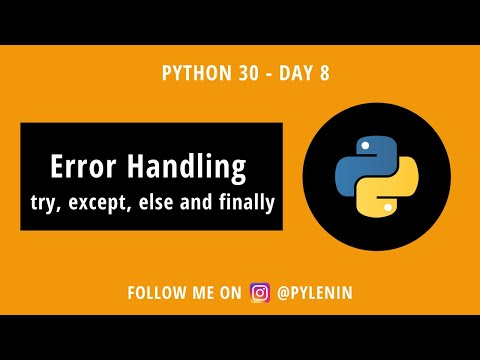# Python Exception Handling: Try, Except, Else and Finally

By Lenin Mishra

This is the 8th topic in the Python 30 series. Check out the series here.

As soon as Python encounters an error, it terminates immediately. An `Exception` event is raised. This affects the flow of the program.

In order to be able to continue with the normal flow, we can use try, except, else and finally keywords to handle the `Exception` class.

#### Types of Errors in Python

Broadly, there are 2 types of errors.

1. Syntax Errors
2. Logical Errors(Exceptions)
##### Syntax Errors

Syntax errors are basically typos in your Python code. They are easy to fix.

Code

``print("Pylenin"))``

Output

``````File "folder-name", line 1
print("Pylenin"))
^
SyntaxError: unmatched ')'``````
##### Logical Errors(Exceptions)

Logical Errors or Exceptions are errors in the logic of a program.

Code

``x = 1/0``

Output

``````Traceback (most recent call last):
File "folder-name", line 1
x = 1/0
ZeroDivisionError: division by zero``````

#### Handling Errors with try, except, else and finally

• try - Tests the code for any error
• except - Catches the error, if any
• else - Only executed, if there is no error
• finally - Always executed#### try-except block

First, let us understand how try and except work.

Code

``````try:
x = 1/0
except:
print("There was some issue")``````

Output

``There was some issue``

As you can see, the program wasn’t interrupted due to the division by zero error. The `print` statement in the `except` block was executed. So if there is no exception, only the code in the `try` block is executed.

Let’s try to find out more info about the exception that happened above. For this purpose, we can use the `Exception` class from python.

Code

``````try:
x = 1/0
except Exception as e:
print(e.__class__)
print(f"Exception occured - {e}")``````

Output

``````<class 'ZeroDivisionError'>
Exception occured - division by zero``````

The `e.__class__` tells us the python built in exception class that was cause of the above error, which is `ZeroDivisionError`.

Let’s try out another example. Now we will try to access an imaginary file that doesn’t exist in our working directory.

Code

``````try:
x = open('imaginary_file.csv', 'r')
except Exception as e:
print(e.__class__)
print(f"Exception occured - {e}")``````

Output

``````<class 'FileNotFoundError'>
Exception occured - [Errno 2] No such file or directory: 'imaginary_file.csv'``````

Now we get the `FileNotFoundError` error. You can find more about built in python exceptions here.

#### Using try except else and finally

We can also include `else` and `finally` keywords into our python code to handle errors.

The code inside the `else` block is only executed if no exceptions are raised.

The code inside the `finally` block is always executed, no matter what!

Let’s look at the following code. Code

``````import logging

log_format = "%(asctime)s::%(levelname)s::%(name)s::"\
"%(filename)s::%(lineno)d::%(message)s"

logging.basicConfig(filename='mylogs.log', level='DEBUG', format=log_format)

def division(x, y):
result = None
try:
result = x/y
except Exception as e:
print(f"Exception - {e} occured")
else:
print(f"The result of {x} divided by {y} is {result}")
finally:
status = None
if not result:
status = "FAIL"
else:
status = "PASS"
logging.info(f"Division function called. RESULT: {status}")

division(3,0)``````

Output

``````#terminal
Exception - division by zero occured

#mylogs.log
2021-01-08 09:08:52,099::INFO::root::trial.py::22::Division function called. RESULT: FAIL``````

As you can see, the code inside the `else` block wasn’t executed. However, the code inside the `finally` block was executed and a log was created in our `mylogs.log` file. The `finally` block is therefore, a great place to log function or class calls.

Let’s change the function call and see what happens.

Code

``````import logging

log_format = "%(asctime)s::%(levelname)s::%(name)s::"\
"%(filename)s::%(lineno)d::%(message)s"

logging.basicConfig(filename='mylogs.log', level='DEBUG', format=log_format)

def division(x, y):
result = None
try:
result = x/y
except Exception as e:
print(f"Exception - {e} occured")
else:
print(f"The result of {x} divided by {y} is {result}")
finally:
status = None
if not result:
status = "FAIL"
else:
status = "PASS"
logging.info(f"Division function called. RESULT: {status}")

division(3,1)``````

Output

``````#terminal
The result of 3 divided by 1 is 3.0

#mylogs.log
2021-01-08 09:13:24,391::INFO::root::trial.py::22::Division function called. RESULT: PASS``````

As you can see, the code inside the `else` block has been executed successfully as there was no error.

Python Logging

If you like to watch Youtube videos over reading blogs, click below.Check out how to work with Python Built-in Exception classes in Python.